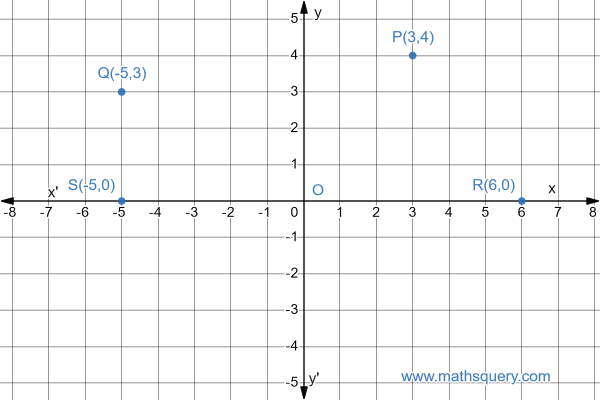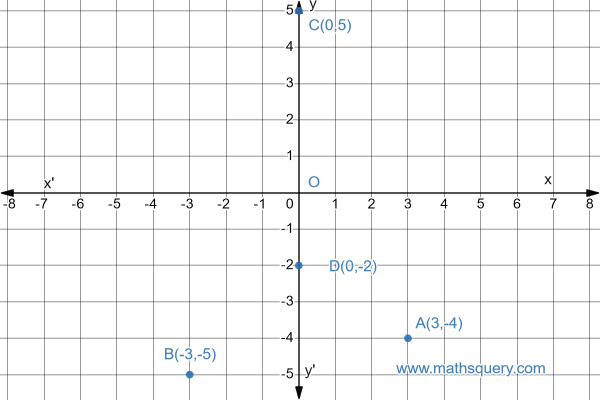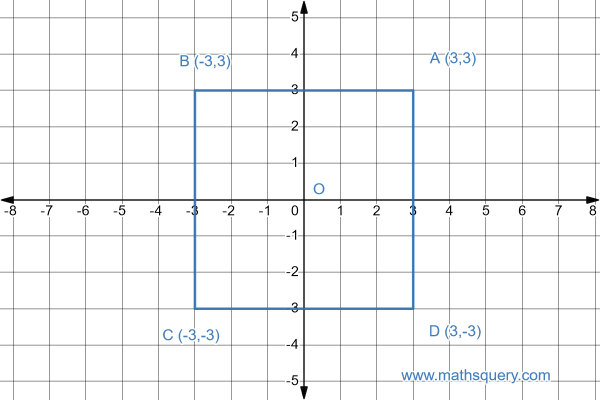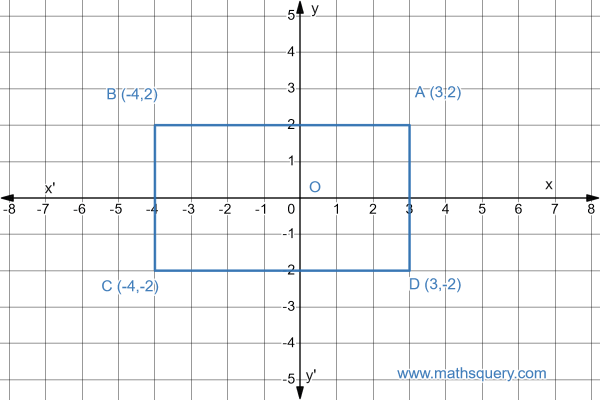MATHS QUERY

# Cartesian Coordinate System

## Introduction

Cartesian Coordinate System is used to describe the position of a point in a plane using coordinates in coordinate geometry. Coordinates are the way of telling the position of a point using numbers.
Rene Discartes invented the Coordinate System and the term Cartesian Coordinate System is named after the name of Discartes mathematician to honour the inventor.
Cartesian geometry system introduces coordinate axes, coordinates and quadrants to describe the position of a point in a plane and its distances from other points.
Let’s learn about these new terms next.

## Coordinate axes

Cartesian coordinate system uses a plane which has two mutually perpendicular lines which are called as coordinate axes. Out of the two perpendicular lines, one line is in horizontal position and another line is in vertical position. The figure below shows how the two coordinate axes look like.

Note

Mind the words axis and axes, how they are used. Axis is used to represent one axis, it is singular. On the other hand, axes is used to represent more than one axis, which is plural.

Horizontal axis in coordinate geometryVertical axis in coordinate geometryThe horizontal line marked with XX is called as x-axis and vertical line marked as YY is called as y-axis. Both axes, x-axis and y-axis intersect each other at point O and are perpendicular to each other. The point O is called as origin.

## Directions of axes

So, x-axis has two sides, one side lies on right side of y-axis and can be read as OX. The second side lies on left side of y-axis and is read as OX. Similarly, y-axis has two sides top and bottom of x-axis. The top side of y-axis is read as OY and bottom side of y-axis is OY’.
The OX on x-axis and OY on y-axis called as positive directions of x-axis and y-axis respectively. Similarly, the OX on x-axis and OY on y-axis called as negative directions of x-axis and y-axis respectively.

Directions of axis in coordinate geometry## Scaling of axes

Scaling of axes is meant by marking the x-axis and y-axis with numbers which are placed at equal distances. The ray OX on x-axis has positive numbers (1, 2, 3, 4, ….) and ray OX has negative numbers (-1, -2, -3, -4, ….) only. Similarly,
The ray OY on y-axis has positive numbers (1, 2, 3, 4, ….) and ray OY has negative numbers (-1, -2, -3, -4, ….) on it. In other words, we can say that positive numbers lie in the directions of ray OX and ray OY and the negative numbers lie in the direction of ray OX and ray OY.

The two coordinate axes x-axis and y-axis in coordinate geometry divide the plane into four parts. These four parts are called as quadrants.In above figure, x-axis and y-axis is dividing the plane into four parts which are XOY, XOY, XOY and XOY.
XOY is called as first quadrant.
XOY is called as second quadrant.
XOY is called as third quadrant.
XOY is called as fourth quadrant.
Also, the four quadrants are numbered as I, II, III and IV anticlockwise starting from first quadrant XOY and the last quadrant as XOY.

## Coordinates of a point

Any point in a plane can lie in any of the four quadrants I, II, III or IV. The position of a point is in the plane is called as coordinates of that point. Coordinates of the point in a quadrant is determined by knowing its perpendicular distances from its nearest x-axis and y-axis.
Coordinates of a point is written by writing its perpendicular distance in brackets in the format of (x,y), where x is the perpendicular distance of the point from x-axis and y is the perpendicular distance of the point from y-axis.
The x-axis coordinate of a point is called as abscissa.
The y-axis coordinate of a point is called as ordinate.
Coordinates of a point can be written only in specific order i.e (x,y). First we write abscissa followed by ordinate and seperated by a comma.

Note

Abscissa and ordinate of a point cannot be interchanged.
i.e. (x,y) ≠ (y,x)
Moreover, (x,y) = (y,x) if x = y

Let’s take an example to understand it precisely

Coordinates of a point in coordinate geometryExample

From the above figure, we can see
P is the point that lie in first quadrant and is marked has P.
The perpendicular distance PN of point P from y-axis is 3 units, measured along the positive direction of x-axis as OM which is 3 units.
The perpendicular distance PM of point P from x-axis is 4 units, measured along the positive direction of y-axis as ON which is 4 units.
So, the perpendicular distance of point P from y-axis is 3 and x-axis is 4, so the coordinate of the point P can be written as (3,4).

Note

Coordinates of origin are always written as (0,0) because perpendicular distance of origin from x-axis is zero and from y-axis is also zero.

Note

The ordinate of any point on x-axis is 0 i.e coordinate of any point on x-axis is (x,0).
The abscissa of any point on y-axis is 0 i.e coordinate of any point on y-axis is (0,y).

As we have read above, the x-axis XOX’ and y-axis YOY’ divide the coordinate plane into four quadrants.
The ray OX is regarded as positive x-axis.
The ray OY is regarded as positive y-axis.
The ray OX is regarded as negative x-axis.
The ray OY is regarded as negative y-axis.
So, any point that lies in first quadrant will always have positive abscissa and positive ordinate.
Any point that lie in second quadrant has negative abscissa and positive ordinate.
Any point that lie in third quadrant has negative abscissa and negative ordinate.
Any point that lie in fourth quadrant has positive abscissa and negative ordinate.
Please refer to the following figure, how the signs of abscissa and ordinate looks like in the four quadrants.

Sign conventions in quadrants in coordinate geometrySo, In the figure:
first quadrant has points with sign (+,+).
second quadrant has points with sign (-,+).
third quadrant has points with sign (-,-).
fourth quadrant has points with sign (+,-).

### Q) What is coordinate geometry?

The branch of mathematics in which coordinate system is used to solve geometric problems is known as coordinate geometry.

### Q) What else is a horizontal line passing through origin called as?

Horizontal line is called as x-axis.

### Q) What else is a vertical line passing through origin called as?

Vertical line is called as y-axis.

### Q) What is called the x coordinate of a point as?

The x coordinate of a point is called as abscissa.

### Q) What is called the y coordinate of a point as?

The y coordinate of a point is called as ordinate.

## Solved Examples

### 1) Plot the points P(3,4), Q(-5,3), R(6,0) and S(-5,0) on the graph paper.Solution

Point P(3,4) lies in the first quadrant. So we move 3 units along OX and 4 units in upward direction i.e along OY.
Point Q(-5,3) lies in second quadrant. So we move 5 units along OX' and 3 units in upward direction i.e along OY.
Point R(-5,0) lies on negative direction of x-axis at the distance of 5 units from origin.
Point S(6,0) lies on positive direction of x-axis at the distance of 6 units from origin.

### 2) Plot the points A(3,-4), B(-3,-5), C(0,5) and D(-0,-2) on the graph paper.

SolutionPoint A(3,-4) lies in fourth quadrant. So we move 3 units along OX and 4 units in downward direction i.e along OY'.
Point B(-3,-5) lies in third quadrant. So we move 3 units along OX' and 5 units in downward direction i.e along OY'.
Point C(0,5) lies on positive direction of y-axis at the distance of 5 units from origin.
Point D(0,-2) lies on negative direction of y-axis at the distance of 2 units from origin.

### 3) Locate the points A(3,3), B(-3,3), C(-3,-3) and D(3,-3) on graph paper. Name the figure by joining these points. Also find its perimeter.Solution

The figure joined by these points is a square.
AB = BC = CD = DA = 6 units
side of square = 6 units
Perimeter of square= 4 x 6 = 24 sq. units

### 4) Plot the points A(3,2), B(-4,2), C(-4,-2) and D(3,-2) on graph paper. Name the figure by joining these points. Also find its area.Solution

AB = CD = 7 units
BC = AD = 4 units
So, ABCD is a rectangle
Area of rectangle = length x breadth
= 7 x 4 = 28 sq. units

### 5) Plot the points M(3,0), N(3,4), and O(0,0) on graph paper. Find area of the figure by joining these points.Solution

The figure is right angled triangle when we join these points.
OM = 3 units
MN = 4 units
∠OMN = 900
Area of $$\triangle OMN$$ = $$\frac{1}{2}$$ x base x height
= $$\frac{1}{2}$$ x OM x MN
= $$\frac{1}{2}$$ x 3 x 4
= 6 sq. units

## Multiple Choice Questions

1. abscissa
2. ordinate
3. origin
4. coordinates

### 2) Which mathematician did develop the cartesian system of coordinates?

1. Thales
2. Euclid
3. Pythagoras
4. Rene descartes

### 3) A Horizontal line and a vertical line in a coordinate plane are called as

1. x-axis and y-axis
2. y-axis and x-axis
3. abscissa and ordinate
4. ordinate and abscissa

### 4) Points (2,5) and (5,2) represent a same point in a cartesian plane.

1. True
2. False
3. Maybe
4. None of these

1. (-4,0)
2. (0,-4)
3. (2,-1)
4. (-1,-2)

1. (+,+)
2. (+,-)
3. (-,+)
4. (-,-)

### 8) Points (x,y) and (y,x) represent a same point in a plane if

1. x < y
2. x > y
3. x = y
4. not possible

### 9) If in cartesian plane, the points (x+3,6) and (-1,y+2) represent a same point, then value of x and y will be

1. x = -4 and y = 4
2. x = -4 and y = -4
3. x = 3 and y = 6
4. x = -1 and y = 6

1. (4,0)
2. (0,4)
3. (-3,1)
4. (-3,-2)